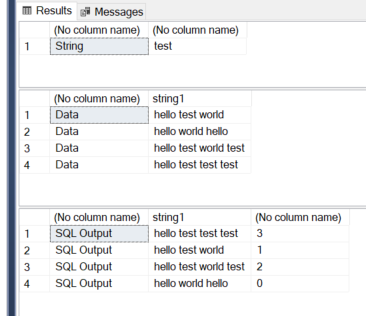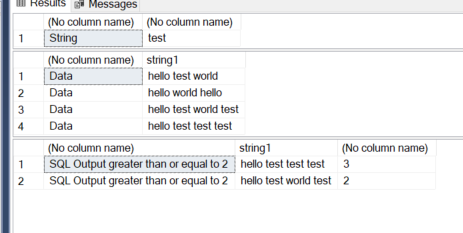# Find rows with duplicate sub strings

Given a table with a column of varchar(max), I want to find all rows have a certain sub string that occurs more than once.

For example:
row 1: hello test world
row 2: hello world hello
row 3: hello test world test
row 3: hello test test test

I want to find all rows that contain the sub string 'test' at least twice, so rows 3 and 4 only in this case.

A simple attempt:

select * from mytable where mycol like '%test%%test%'

No real idea how to proceed beyond that.

Any help appreciated!

hi

there are several ways to do this

approaches

hi

one way is using XML method .. hope this helps``````;WITH [cte]
AS (
SELECT
string1
, LTRIM(RTRIM([m].[n].[value]('.', 'varchar(8000)'))) AS [string_split]
FROM
(   SELECT
string1
, CAST('<XMLRoot><RowData>' + REPLACE(#temp.string1, 'test', '</RowData><RowData>')
+ '</RowData></XMLRoot>' AS XML) AS [x]
FROM
#temp) AS [t]
CROSS APPLY [x].[nodes]('/XMLRoot/RowData') AS [m]([n])
)
select 'SQL Output',string1,count(*)-1 from   [cte]
group by string1
````````````drop table #temp

create table #temp
(
string1  VARCHAR(200)
)

insert into #temp select 'hello test world'
insert into #temp select 'hello world hello'
insert into #temp select 'hello test world test'
insert into #temp select 'hello test test test'
``````

declare @string varchar(200) = 'test'

select 'String',@string

select 'Data',* from #temp

``````;WITH [cte]
AS (
SELECT
string1
, LTRIM(RTRIM([m].[n].[value]('.', 'varchar(8000)'))) AS [string_split]
FROM
(   SELECT
string1
, CAST('<XMLRoot><RowData>' + REPLACE(#temp.string1, 'test', '</RowData><RowData>')
+ '</RowData></XMLRoot>' AS XML) AS [x]
FROM
#temp) AS [t]
CROSS APPLY [x].[nodes]('/XMLRoot/RowData') AS [m]([n])
)
select 'SQL Output greater than or equal to 2',string1,count(*)-1 from   [cte]
group by string1
having count(*)-1 >= 2
``````another way

``````select distinct string1
from (
select ROW_NUMBER() OVER (
PARTITION BY string1
ORDER BY string1
) row_num, *
from #temp
cross apply string_split(string1, ' ') a
where value = @string
) a
where row_num >= 2
``````

``````DECLARE @number_of_string_matches_required smallint
DECLARE @string_to_find varchar(200)

SET @string_to_find = 'test'
SET @number_of_string_matches_required = 2

;WITH cte_test_data AS (
SELECT * FROM (VALUES
(1, 'hello test world'),
(2, 'hello world hello'),
(3, 'hello test world test'),
(4, 'hello test test test'))
AS data(row#, string)
)
SELECT *
FROM cte_test_data
WHERE (LEN(string) - LEN(REPLACE(string, @string_to_find, ''))) /
LEN(@string_to_find) >= @number_of_string_matches_required
ORDER BY row#``````
2 Likes

hi Scott

I like your way .... had something like this in mind .. but could not implementFascinating!

Easy to remember trick to find nth number of occurrence of a string.

Love you.

Thanks! Yep, it comes in very handy at times.